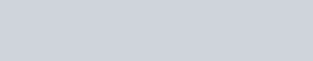# Python SciKit Learn Tutorial

Filed Under: Machine Learning

## Scikit LearnScikit-learn is a machine learning library for Python. It features several regression, classification and clustering algorithms including SVMs, gradient boosting, k-means, random forests and DBSCAN. It is designed to work with Python Numpy and SciPy.

The scikit-learn project kicked off as a Google Summer of Code (also known as GSoC) project by David Cournapeau as scikits.learn. It gets its name from “Scikit”, a separate third-party extension to SciPy.

## Python Scikit-learn

Scikit is written in Python (most of it) and some of its core algorithms are written in Cython for even better performance.

Scikit-learn is used to build models and it is not recommended to use it for reading, manipulating and summarizing data as there are better frameworks available for the purpose.

It is open source and released under BSD license.

### Install Scikit Learn

Scikit assumes you have a running Python 2.7 or above platform with NumPY (1.8.2 and above) and SciPY (0.13.3 and above) packages on your device. Once we have these packages installed we can proceed with the installation.

For pip installation, run the following command in the terminal:

``````
pip install scikit-learn
``````

If you like `conda`, you can also use the conda for package installation, run the following command:

``````
conda install scikit-learn
``````

## Using Scikit-Learn

Once you are done with the installation, you can use scikit-learn easily in your Python code by importing it as:

``````
import sklearn
``````

Let’s start with loading a dataset to play with. Let’s load a simple dataset named Iris. It is a dataset of a flower, it contains 150 observations about different measurements of the flower. Let’s see how to load the dataset using scikit-learn.

``````
# Import scikit learn
from sklearn import datasets
# Print shape of data to confirm data is loaded
print(iris.data.shape)
``````

We are printing shape of data for ease, you can also print whole data if you wish so, running the codes gives an output like this:### Scikit Learn SVM – Learning and Predicting

Now we have loaded data, let’s try learning from it and predict on new data. For this purpose we have to create an estimator and then call its fit method.

``````
from sklearn import svm
from sklearn import datasets
clf = svm.LinearSVC()
# learn from the data
clf.fit(iris.data, iris.target)
# predict for unseen data
clf.predict([[ 5.0,  3.6,  1.3,  0.25]])
# Parameters of model can be changed by using the attributes ending with an underscore
print(clf.coef_ )
``````

Here is what we get when we run this script:### Scikit Learn Linear Regression

Creating various models is rather simple using scikit-learn. Let’s start with a simple example of regression.

``````
#import the model
from sklearn import linear_model
reg = linear_model.LinearRegression()
# use it to fit a data
reg.fit ([[0, 0], [1, 1], [2, 2]], [0, 1, 2])
# Let's look into the fitted data
print(reg.coef_)
``````

Running the model should return a point that can be plotted on the same line:### k-Nearest neighbour classifier

Let’s try a simple classification algorithm. This classifier uses an algorithm based on ball trees to represent the training samples.

``````
from sklearn import datasets
# Create and fit a nearest-neighbor classifier
from sklearn import neighbors
knn = neighbors.KNeighborsClassifier()
knn.fit(iris.data, iris.target)
# Predict and print the result
result=knn.predict([[0.1, 0.2, 0.3, 0.4]])
print(result)
``````

Let’s run the classifier and check results, the classifier should return 0. Let’s try the example:### K-means clustering

This is the simplest clustering algorithm. The set is divided into ‘k’ clusters and each observation is assigned to a cluster. This is done iteratively until the clusters converge.

We will create one such clustering model in the following program:

``````
from sklearn import cluster, datasets
# create clusters for k=3
k=3
k_means = cluster.KMeans(k)
# fit data
k_means.fit(iris.data)
# print results
print( k_means.labels_[::10])
print( iris.target[::10])
``````

On running the program we’ll see separate clusters in the list. Here is the output for above code snippet:## Conclusion

In this tutorial, we have seen that Scikit-Learn makes it easy to work with several machine learning algorithms. We have seen examples of Regression, Classification and Clustering.

Scikit-Learn is still in development phase and being developed and maintained by volunteers but is very popular in community. Go and try your own examples.

1.Sid says:

Hi,.. While installing i am getting the following error

rom distutils
customize MSVCCompiler
Missing compiler_cxx fix for MSVCCompiler
customize MSVCCompiler using build_clib
building ‘libsvm-skl’ library
compiling C sources
error: Microsoft Visual C++ 14.0 is required. Get it with “Microsoft Visual
C++ Build Tools”: `https://landinghub.visualstudio.com/visual-cpp-build-tools`

—————————————-
Command “c:\users\sidtrive\appdata\local\programs\python\python37-32\python.exe
-u -c “import setuptools, tokenize;__file__=’C:\\Users\\sidtrive\\AppData\\Local
\\Temp\\pip-install-2rnp9ekh\\scikit-learn\\setup.py’;f=getattr(tokenize, ‘open’